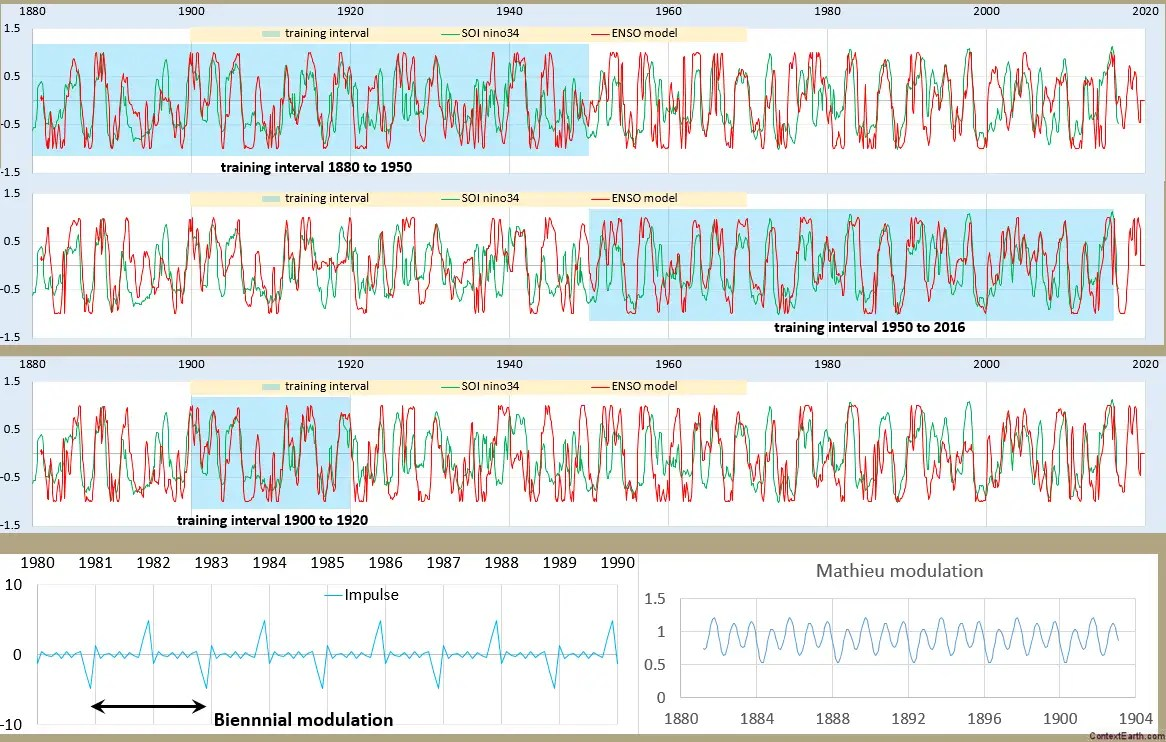# Canonical Solution of Mathieu Equation for ENSO

[mathjax]
From a previous post, we were exploring possible solutions to the Mathieu equation given a pulsed stimulus.  This is a more straightforward decomposition of the differential equation using a spreadsheet.

The Mathieu equation:$f''(t) + omega_0^2 (1 + alpha cos(nu t)) f(t) = F(t)$

can be approximated as a difference equation, where the second derivative f”(t) is ~ (f(t)-2f(t-dt)+f(t-2dt))/dt. But perhaps what we really want is a difference to the previous year and determine if that is enough to reinforce the biennial modulation that we are seeing in the ENSO behavior.

Setting up a spreadsheet with a lag term and a 1-year-prior feedback term, we apply both the biennial impulse-modulated lunar forcing stimulus and a yearly-modulated Mathieu term.Fig. 1: Training (in shaded blue) and test for different intervals.

I was surprised by how remarkable the approximate fit was in the recent post, but this more canonical analysis is even more telling. The number of degrees of freedom in the dozen lunar amplitude terms apparently has no impact on over-fitting, even on the shortest interval in the third chart. There is noise in the ENSO data no doubt, but that noise seems to be secondary considering how the fit seems to mostly capture the real signal. The first two charts are complementary in that regard — the fit is arguably better in each of the training intervals yet the test interval results aren’t really that much different from the direct fit looking at it by eye.

Just like in ocean tidal analysis, the strongest tidal cycles dominate;  in this case the Draconic and Anomalistic monthly, the Draconic and Anomalistic fortnightly, and a Draconic monthly+Anomalistic fortnightly cross term are the strongest (described here). Even though there is much room for weighting these factors differently on orthogonal intervals, the Excel Solver fit hones in on nearly the same weighted set on each interval.  As I said in a previous post, the number of degrees of freedom apparently do not lead to over-fitting issues.

One other feature of this fit was an application of a sin() function applied to the result. This is derived from the Sturm-Liouville solution to Laplace’s tidal equation used in the QBO analysis  — which works effectively to normalize the model to the data, since the correlation coefficient optimizing metric does not scale the result automatically.

Pondering for a moment, perhaps the calculus is not so different to work out after all:

A bottom-line finding is that there is really not much complexity to this unique tidal formulation model of ENSO.  But because of the uniqueness of the seasonal modulation that we apply, it just doesn’t look like the more-or-less regular cycles contributing to sea-level height tidal data.  Essentially similar algorithms are applied to find the right weighting of tidal factors, but whereas the SLH tidal data shows up in daily readings, the ENSO data is year-to-year.

Further, the algorithm does not take more than a minute or two to finish fitting the model to the data. Below is a time lapse of one such trial. Although this isn’t an optimal fit, one can see how the training interval solver adjustment (in the shaded region) pulls the rest of the modeled time-series into alignment with the out-of-band test interval data.## 3 thoughts on “Canonical Solution of Mathieu Equation for ENSO”

1. Pingback: Scaling El Nino | context/Earth